# GMAT Math : DSQ: Graphing a quadratic function

## Example Questions

2 Next →

### Example Question #41 : Graphing

How many-intercepts does a vertical parabola on the coordinate plane have—zero, one, or two?

Statement 1: The parabola intersects the graph of the equation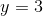twice.

Statement 2: The parabola has-intercept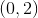.

BOTH statements TOGETHER are sufficient to answer the question, but NEITHER statement ALONE is sufficient to answer the question.

EITHER statement ALONE is sufficient to answer the question.

Statement 1 ALONE is sufficient to answer the question, but Statement 2 ALONE is NOT sufficient to answer the question.

BOTH statements TOGETHER are insufficient to answer the question.

Statement 2 ALONE is sufficient to answer the question, but Statement 1 ALONE is NOT sufficient to answer the question.

BOTH statements TOGETHER are insufficient to answer the question.

Explanation:

Assume both statements are true, and consider these two equations:

Case 1: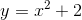The-intercept can be proven to beby substituting 0 for: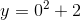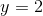We show that the graph intersects the line of equationtwice by substituting 3 for: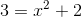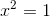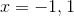The points of intersection are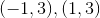.

To find the-intercept(s), if any exist, substitute 0 for: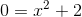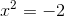This has no real solutions, so the parabola has no-intercepts.

Case 2: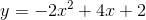The-intercept be proved to beby substituting 0 for: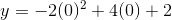We show that the graph intersects the line of equationtwice by substituting 3 for: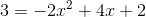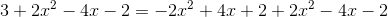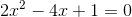We examine the discriminant: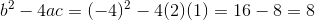The discriminant is positive, so there are two real solutions, meaning that there are two points of intersection.

To find the-intercept(s), if any exist, substitute 0 for: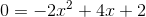We examine this discriminant: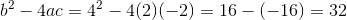The discriminant is positive, so there are two real solutions, meaning that there are two-intercepts.

Two parabolas have been identified fitting the main condition and those of both statements, but one has no-intercept and one has two. The two statements together provide insufficient information.

### Example Question #561 : Geometry

What is the equation of the line of symmetry of a vertical parabola on the coordinate plane?

Statement 1: The parabola has one of its two-intercepts at the point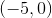.

Statement 2: The-intercept of the parabola is at the origin.

Statement 2 ALONE is sufficient to answer the question, but Statement 1 ALONE is NOT sufficient to answer the question.

Statement 1 ALONE is sufficient to answer the question, but Statement 2 ALONE is NOT sufficient to answer the question.

EITHER statement ALONE is sufficient to answer the question.

BOTH statements TOGETHER are insufficient to answer the ques

BOTH statements TOGETHER are sufficient to answer the question, but NEITHER statement ALONE is sufficient to answer the question.tion.

BOTH statements TOGETHER are sufficient to answer the question, but NEITHER statement ALONE is sufficient to answer the question.tion.

Explanation:

The line of symmetry of a vertical parabola is the vertical line passing through the vertex. Each statement alone gives only one point on the graph, neither of which is the vertex, so neither statement alone gives sufficient information.

Now assume both statements to be true. Statement 1 gives one-intercept,; Statement 2 states that the graph passes through the origin, so it is not only the-intercept, it is also the other-intercept. The-coordinate of the vertex is the arithmetic mean of those of the two-intercepts, so that value is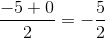Only the-coordinate of the vertex is needed to answer the question - we can immediately deduce that the line of symmetry is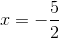.

### Example Question #43 : Graphing

The equation of a vertical parabola on the coordinate plane can be written in the form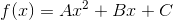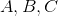real,nonzero.

Is this parabola concave upward or concave downward?

Statement 1: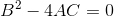Statement 2: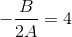BOTH statements TOGETHER are insufficient to answer the question.

BOTH statements TOGETHER are sufficient to answer the question, but NEITHER statement ALONE is sufficient to answer the question.

Statement 2 ALONE is sufficient to answer the question, but Statement 1 ALONE is NOT sufficient to answer the question.

Statement 1 ALONE is sufficient to answer the question, but Statement 2 ALONE is NOT sufficient to answer the question.

EITHER statement ALONE is sufficient to answer the question.

BOTH statements TOGETHER are insufficient to answer the question.

Explanation:

The parabola is concave upward if and only if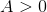, and concave downward if and only if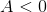. Therefore, we need to know the sign ofto answer the question. We show that the two statements together provide insufficient information by examining two equations.

Case 1: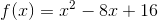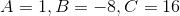; we check the values of the expressions in both statements: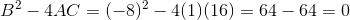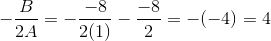The conditions of both statements are satisfied; since, the parabola that graphs this function is concave upward.

Case 2: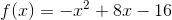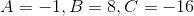; we check the values of the expressions in both statements: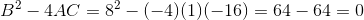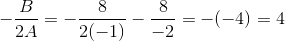The conditions of both statements are satisfied; since, the parabola that graphs this function is concave downward.

### Example Question #2791 : Gmat Quantitative Reasoning

What is the equation of the line of symmetry of a parabola on the coordinate plane?

Statement 1: The vertex of the parabola has-coordinate.

Statement 2: The vertex of the parabola has-coordinate.

EITHER statement ALONE is sufficient to answer the question.

BOTH statements TOGETHER are insufficient to answer the question.

Statement 1 ALONE is sufficient to answer the question, but Statement 2 ALONE is NOT sufficient to answer the question.

Statement 2 ALONE is sufficient to answer the question, but Statement 1 ALONE is NOT sufficient to answer the question.

BOTH statements TOGETHER are sufficient to answer the question, but NEITHER statement ALONE is sufficient to answer the question.

BOTH statements TOGETHER are insufficient to answer the question.

Explanation:

The two statements together give the vertex of the parabola, but no clue is given as to the orientation of the parabola - horizontal, vertical, or otherwise. Without that information, or any way to find it, the line of symmetry cannot be determined.

### Example Question #45 : Graphing

The equation of a vertical parabola on the coordinate plane can be written in the formwherereal, andis a nonzero number.

Is this parabola concave upward or concave downward?

Statement 1: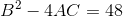.

Statement 2: The parabola has-intercept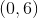.

Statement 2 ALONE is sufficient to answer the question, but Statement 1 ALONE is NOT sufficient to answer the question.

EITHER statement ALONE is sufficient to answer the question.

BOTH statements TOGETHER are sufficient to answer the question, but NEITHER statement ALONE is sufficient to answer the question.

BOTH statements TOGETHER are insufficient to answer the question.

Statement 1 ALONE is sufficient to answer the question, but Statement 2 ALONE is NOT sufficient to answer the question.

BOTH statements TOGETHER are insufficient to answer the question.

Explanation:

Assume both statements are true.

The parabola is concave upward if and only if, and concave downward if and only if. Therefore, we need to know the sign ofto answer the question. We show that the two statements together provide insufficient information by examining two equations.

Case 1: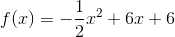If we substitute 0 foris easily seen to be equal to 6, so the-intercept is. Also: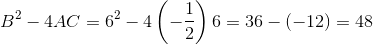Case 2: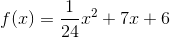If we substitute 0 foris easily seen to be equal to 6, so the-intercept is. Also: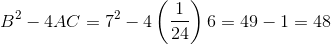Each equation satisfies the conditions of both statements, butis positive in one equation and negative in the other.can assume either sign, so the question of the parabola's concavity is unresolved.

2 Next →

### All GMAT Math Resources